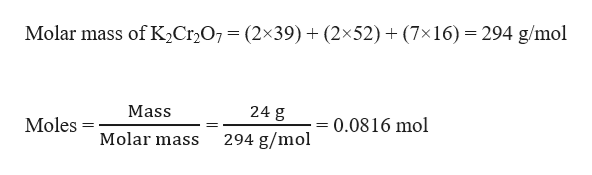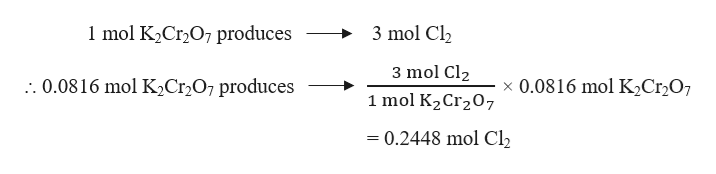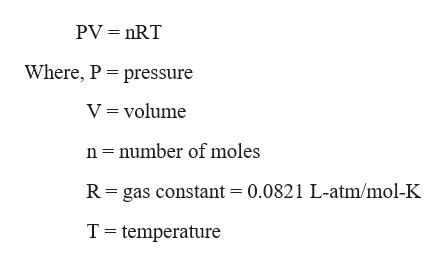# How many liters of Cl2(g) at STP can be made from NaCl by oxidation with 24.0 grams of K2Cr2O7 in an acidic solution after chromium is reduced to Cr3+. 6NaCl + K2Cr2O7 + 7H2SO4 = 3Cl2 + Cr2(SO4)3 + 3Na2SO4 + K2SO4+ 7H2O

Question
26 views

How many liters of Cl2(g) at STP can be made from NaCl by oxidation with 24.0 grams of K2Cr2O7 in an acidic solution after chromium is reduced to Cr3+

6NaCl + K2Cr2O7 + 7H2SO4 = 3Cl2 + Cr2(SO4)3 + 3Na2SO4 + K2SO4+ 7H2

check_circle

Step 1

Given,

Grams of K2Cr2O7 = 24.0 g

Moles of K2Cr2O7 can be calculated as:help_outlineImage Transcriptionclose294 g/mol Molar mass of K2Cr2O7= (2x39) + (2x52)+ (7x16) 24 g Mass 0.0816 mol Moles 294 g/mol Molar mass fullscreen
Step 2

The balanced chemical reaction is given as:

6 NaCl + K2Cr2O7 + 7 H2SO4 → 3 Cl2 + Cr2(SO4)3 + 3 Na2SO4 + K2SO4+ 7 H2

From the reaction, it is evident that:help_outlineImage Transcriptionclose1 mol K2Cr2O7 produces 3 mol Cl2 3 mol Cl2 :. 0.0816 mol K2Cr2O7 produces x 0.0816 mol K2Cr2O7 1 mol K2Cr207 - 0.2448 mol Cl2 fullscreen
Step 3

The ideal gas equatio...help_outlineImage TranscriptionclosePV nRT Where, P pressure V = volume n number of moles R gas constant = 0.0821 L-atm/mol-K T temperature fullscreen

### Want to see the full answer?

See Solution

#### Want to see this answer and more?

Solutions are written by subject experts who are available 24/7. Questions are typically answered within 1 hour.*

See Solution
*Response times may vary by subject and question.
Tagged in

### Chemistry